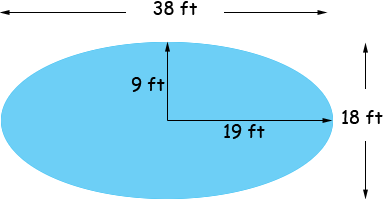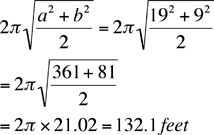Name: Troy Who is asking: Teacher Level of the question: Secondary Question: How many Linear feet would a pool be if the pool was 18 by 38 foot oval. Troy, I am going to assume that your oval is an ellipse.Unfortunately there is no simple expression for the perimeter of an ellipse, but there is an approximation that you can find in a solution to an earlier problem. To use this approximation you need the lengths of the semi-axes a and b of the ellipse. In your situation a = 19 ft and b = 9 ft. The approximation is to the perimeter isPenny